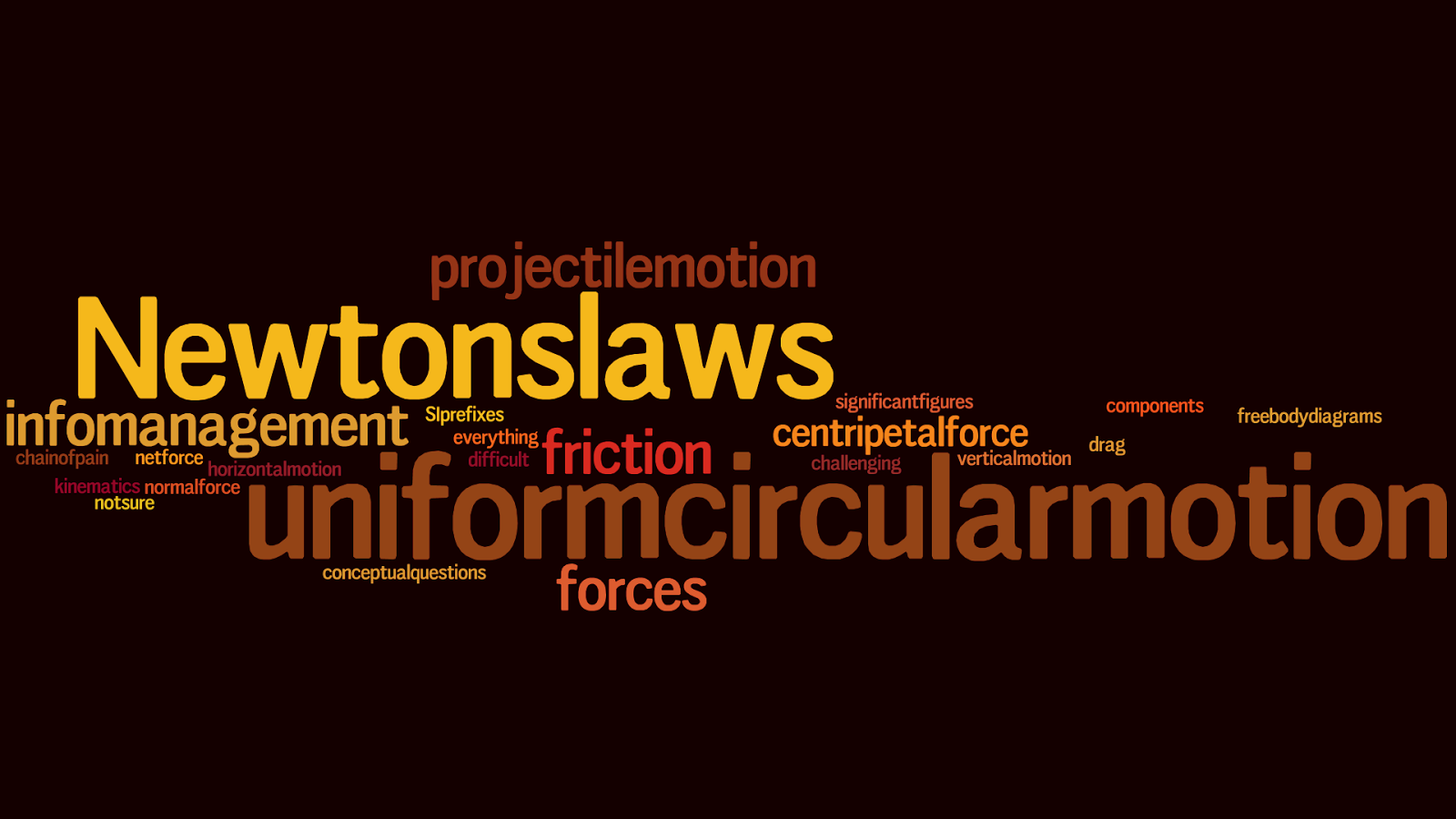## 20171020

### Online reading assignment: confusing Midterm 1 physics topics

Physics 205A, fall semester 2017
Cuesta College, San Luis Obispo, CA"2017-07 Phys 205A confusing"
Waifer X
flic.kr/p/ZC7p5K

Wordle.net tag cloud for confusing topics covered in Midterm 1, generated by responses from Physics 205A students at Cuesta College, San Luis Obispo, CA.

Students have a bi-weekly online reading assignment (hosted by SurveyMonkey.com), where they answer questions based on reading their textbook, material covered in previous lectures, opinion questions, and/or asking (anonymous) questions or making (anonymous) comments. Full credit is given for completing the online reading assignment before next week's lecture, regardless if whether their answers are correct/incorrect. Selected results/questions/comments are addressed by the instructor at the start of the following lecture.

List at least three words describing confusing subjects covered in class (up through this midterm). (Graded for completion.)

These raw responses were subsequently edited to consolidate related common subjects.
Uniform Circular motion, is by far the most confusing
circular motion problems
Again, not sure what this is asking.
Calculus chain of pain
Horizontal vs. vertical, truncating-to-one-uncertain-sig-fig
Circular motion was tricky to grasp but I am figuring it out now.
Uniform circular motion
Uniform circular motion
newtons laws, forces, projectile motion
figuring out the best equation to use; assuming things that aren't stated, and just word play when talking about forces acting upon objects. Sometimes I think that could have been worded differently to make it less confusing.
Air resistance, circular motion, normal force free body diagram
To be honest I'm a little lost on the whole concept.
challenging, difficult, a lot of information
stubborn Newtons's laws, jumbled constant acceleration equations, and plentiful SI prefixes
sums, centripetal, stiction
Projectile motion, Newton's third law was a little confusing in the beginning, static friction was a little confusing as well.
Newton's second law
Newton's laws, static, kinetic
Describing the forces, uniform circular motion
Newton's laws applications
Projectile motion, velocity components
Centripetal force is something that I am having a lot of trouble on. I don't get the core concept nor am I able to do example problems relating to it.
Wording Newton's laws, understanding the questions that ask for reasoning rather than solving problems."
Describe your most confusing subject, and briefly explain why this subject confused you. (Graded for completion.)

The following are all of the student responses to this question, verbatim and unedited.
"I think uniform circular motion is the most confusing because, it is something I had never learned before and it combines a bunch of different ways in which the forces act."

"Circular motion is the most confusing because we did not spend much time on it."

"How to apply everything in a situation."

"Newton's third law, I have trouble with how it differs from Newton's first law."

"The difference between horizontal and vertical velocities. It took me a bit to fully understand this concept."

"Circular motion and the forces that are involved seemed to be the hardest part of this midterm material. From real-life experiences, I would have though the forces would be the opposite of what they actually are mathematically."

"Free body diagrams for uniform circular motion or really most motions get really confusing for me and I get lost on where to go with them."

"I struggled understanding the sum of forces for uniform circular motion because I did not understand how they differed."

"Newton's laws were confusing because the first law and the third law are hard to distinguish for me."

"When I look at the problems and use common sense and can figure out which law or object is less or more, I know the answer but it's hard for me to explain why I know that's the answer."

"I am not entirely sure why, but the concept of circular motion was a hard one for me understand. It was confusing to me that the arrows would all be pointing inward on a diagram."

"I'm just not that good at drawing free body diagrams. "

"I'm a little rusty on Newton's laws."

"The different frictions are the most confusing to me because I get lost when we start talking about the applied forces and how many newtons it takes to get something to slide."

"The most confusing was friction force at the beginning as well as uniform circular motion."

"For me, it was the centripetal force and that the sum of the forces point inward."

"Projectile motion was the most confusing for me until I really looked into my trigonometry again since it has been a few years since I took trigonometry."

"Newton's second law because actually knowing when to use it instead of Newton's first law is still difficult for me for some reason, and I don't even know why."

"I'm still so 'stuck' on static and kinetic forces; no matter how much I try and conceptualize it, I can't for some reason. I understand the basics, but I'm worried it won't be enough to be acceptable on the exam."

"Uniform circular motion, because I have a hard explaining why the forces are the way that they are like comparing normal forces in two different cases."

"Newton's laws, I feel I understand the concepts just when it comes to applying all of them I sometimes put them in the wrong spot."

"I think I am going to have the hardest time with projectile motion because it just has a lot of concepts to remember and steps to take when solving problems. It's a little harder to visualize for me."

"Centripetal force makes no sense to me and I am very worried about it on the midterm."

"Often we use Newton's first law, but when it comes to Newton's third law, I am always doubting if I look at the right forces to answer 'POF-OST-ITO.'"